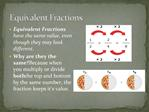DownloadDownload PresentationEquivalent Fractions

# Equivalent Fractions

Télécharger la présentation## Equivalent Fractions

- - - - - - - - - - - - - - - - - - - - - - - - - - - E N D - - - - - - - - - - - - - - - - - - - - - - - - - - -
##### Presentation Transcript

1. Equivalent Fractions Equivalent Fractions have the same value, even though they may look different. Why are they the same? Because when you multiply or divide both the top and bottom by the same number, the fraction keeps it's value.

2. Simplifying Fractions To simplify a fraction, divide the top and bottom by the highest number that can divide into both numbers exactly. Simplifying (or reducing) fractions means to make the fraction as simple as possible.

3. Least Common Denominator (LCD) ..is the LEAST COMMON MULTIPLE of denominators LCM is The smallest (non-zero) number that is a multiple of two or more numbers.

4. Adding Fractions There are 3 Simple Steps to add fractions: Step 1: Make sure the bottom numbers (the denominators) are the same Step 2: Add the top numbers (the numerators). Put the answer over the same denominator as in step 1 Step 3: Simplify the fraction (if needed).

5. Subtracting Fractions There are 3 simple steps to subtract fractions Step 1. Make sure the bottom numbers (the denominators) are the same Step 2. Subtract the top numbers (the numerators). Put the answer over the the same denominator as in step 1 Step 3. Simplify the fraction. �

6. Types of Fractions Proper Fractions Improper Fractions Mixed Numbers

7. Proper Fractions A Proper Fraction has a numerator (top number) less than its denominator (bottom number)

8. Improper Fraction An Improper fraction has a numerator (top number) larger than or equal to the denominator (bottom number)

9. Mixed Numbers A Mixed Fraction is a whole number and a fraction combined

10. Changing Improper to Mixed Fractions Numerator denominator

11. Changing Mixed to Improper Fractions Whole number numerator denominator